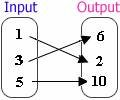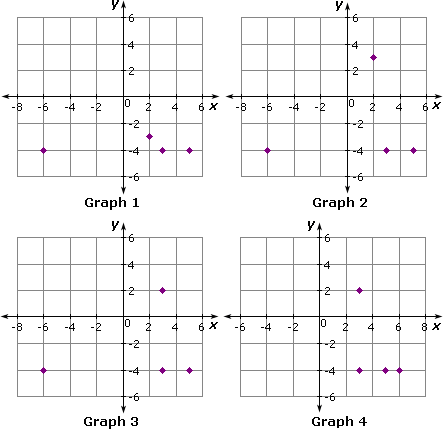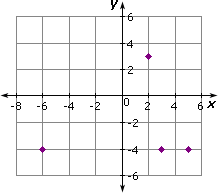## Definition Of Relation

A set of input and output values, usually represented in ordered pairs, refers to a Relation.

Relation is generally represented by a mapping diagram and graph

### Example of Relation

The mapping diagram of the relation {(1, 2), (3, 6), (5, 10)} is shown below.### Solved Example on Relation

#### Ques: Choose a graph that represents the relation {(3, - 4), (5, - 4), (- 6, - 4), (2, 3)}.##### Choices:

A. Graph 3
B. Graph 1
C. Graph 4
D. Graph 2